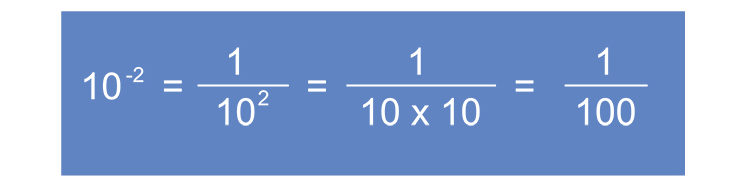# Standard form divide

To divide in standard form all you need to remember is thatNOTE:

See indices Mammoth memory for further explanation

But the basis of divide in standard form is first look for a simple comparable that you know with indices.

i.e. try simple numbers you know first

Example 1

Work out the following giving your answer in standard form

44800div6.4times10^9

## Method 1

Actually carry out the division

44800div6.4times10^9

is the same as

44800div6.4times10times10times10times10times10times10times10times10times10

which is

44800div6,400,000,000

which is

(448cancel0cancel0)/(6,400,000,0cancel0cancel0)

448/(64,000,000)

=0.000007

and to put this in standard form

remember move decimal right and -1 to the power

Answer: is =7times10^-6

## Method 2

An alternative way to calculate this is to say what is a simpler calculation, what is

10^2div10^3

=(cancel10timescancel10)/(10timescancel10timescancel10)=1/10

=10^-1

Therefore

44800div6.4times10^9

is the same as

4.48times10^4div6.4times10^9

(4.48times10^4)/(6.4times10^9)

4.48/6.4times10^4/10^9

0.7times10^-5

and in standard form this is

remember move decimal right and -1 to the power

Answer: is 7times10^-6

Example 2

(1.4times10^12)div(3.2times10^4)

## Method 1

Actually carry out the division

1.4times10^12=1.4times10times10times10times10times10times10

times10times10times10times10times10times10

=1,400,000,000,000

and

3.2times10^4=3.2times10times10times10times10

=32,000

So the calculation becomes

(1,400,000,000,cancel(000))/(32,cancel(000))

=(1,400,000,000)/32

=43,750,000

and to put this in standard form

remember move decimal place left and +1 to the power

Answer is =4.375times10^7

## Method 2

An alternative way to calculate this is to say what is a simpler calculation, what is

10^2div10^3

=(cancel10timescancel10)/(10timescancel10timescancel10)=1/10=10^-1

Therefore:

(1.4times10^12)div(3.2times10^4)

is the same as

(1.4times10^(cancel(\ 12)8))/(3.2timescancel10^cancel(\ 4))

(1.4times10^8)/3.2

=0.4375times10^8

remember move decimal place to right and -1 to the power

and to put this in standard form this becomes

4.375times10^7

Example 3

(3.8times10^8)div(1.9times10^-3)

## Method 1

Actually carry out the division

(3.8times10^8)div(1.9times10^-3)

is the same as

3.8times10times10times10times10times10times10times10times10div1.9/(10times10times10)

which is

380,000,000div0.0019

which is

(380,000,000)/0.0019

=200,000,000,000

and to put this in standard form

remember to move decimal place left and +1 to the power

Answer: is 2times10^11

## Method 2

An alternative is to find a similar calculation that you know. In this case

10^2div10^-2

=1000div0.1

=1000/0.1=10,000

or similarly

10^2div10^-2

is

10^2div1/10^2

=10^2/(1/10^2)

=10^2times10^2

=10^4

=10,000

Now to the actual calculation

(3.8times10^8)div(1.9times10^-3)

would be

(3.8times10^8)div(1.9/10^3)

=(3.8times10^8)/(1.9/10^3)

=(3.8times10^8times10^3)/1.9

=(3.8times10^11)/1.9

=2times10^11

which is already in standard form so the answer is =2times10^11